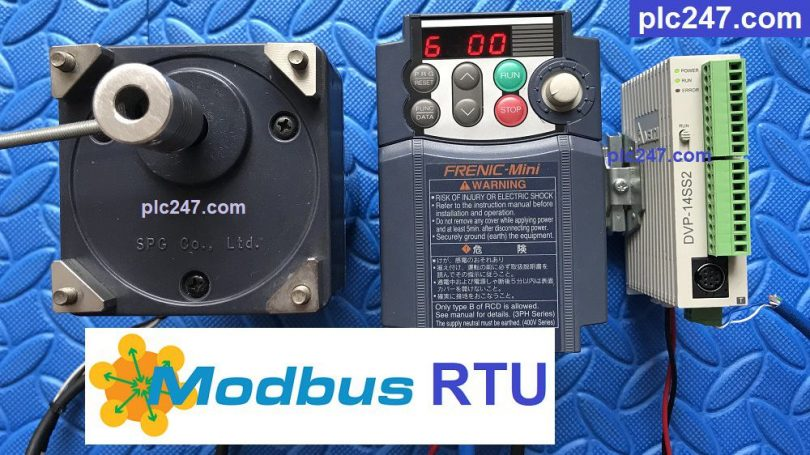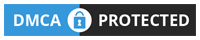# DELTA DVP 14SS2 “Modbus RTU” Fuji Frenic TutorialWritten by

Hello!
In the previous post, plc247.com showed you how to use the Delta DVP-Series PLC to control the Mitsubishi FR-E700 Seires inverter via Modbus-RTU RS485 communication.

Today I would like to continue to guide you to use Delta PLC to control Fuji Frenic inverters (Mini, Eco, Mega, Multi… Series).

#### DVP 14SS2 Modbus-RTU “Wiring Diagram”

Note: If the communication distance is long (>50m), then a 120-Ohm . terminal resistor should be used

#### Fuji Frenic VFD Modbus Configuration

In addition to the basic parameters of the inverter, we need to set the communication parameters as follows:

+ H30 = 3 (Control via RS485 Port)

+ Y01 = 1 ( Slave No.1)

+ Y02 = 0

+ Y03 = 2

+ Y04 = 2 (9600bps)

+ Y05 = 0 (bit)

+ Y06 = 1 (Even)

+ Y07 = 1 ( Stopbit-1)

+ Y10 = 0 (Choose Modbus-RTU)

+ Y99 = 0

Looking at the table above we can see:

+ Frequency Reference = S(07Hex) + 05 = 705 (Hexa)

+ Operation Command = S(07Hex) + 06 = 706 (Hexa)

• Set 706 (Hex) = 1 >>> Motor Run Forward
= 2 >>> Motor Run Reverse
= 0 >>> Motor Stop

+ Output Frequency Address = M (08H) + 09 = 809 (Hex)

+ Input Power Address = 80A (Hex)

+ Output Current Address = 80B (Hex)

+ Output Voltage Address = 80C (Hex)

#### PLC Delta Modbus-RTU Configurator

We need to set the same communication parameters as the inverter (8.1,Even).

The software will automatically create the program as below:

#### PLC Programming

+ Frequency Setting Command

+ Control Motor Command

### Video Running Program

======

Required Documentation and Software:

+ Fuji Frenic Parameters Setting Manual PDF (Password Extract: plc247.com)
Fuji Frenic Series Modbus RS485 Manual PDF

If you have any difficulty in connecting PLC Delta DVP 14SS2 with Fuji Frenic inverter, please comment below the article, plc247.com will reply to you as soon as I read it.

Best Regards!#### plc247.com

•Peppino says:
•plc247.com says: